In winters, you hear chattering sound while taking off your sweater vest. Well, these are the sparks created by electric charges.

Notice this next time if you haven’t already.

Electric charges are all around us. Just like gravity exerts a force on all the objects having mass, charges also exert force on other charges.

Electrostatic force depends on the two charges as well as the distance between them.

Coulomb’s law: It states that the electrical force between two charged objects is directly proportional to the product of the quantity of charge on the objects and inversely proportional to the square of the separation distance between the two objects.

Mathematically, it is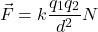where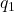represents the charge 1 (in Coulombs),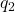represents the charge 2 (in Coulombs), and d represents the distance of separation between the two objects (in meters).

The symbol k is a proportionality constant known as the Coulomb’s law constant.Where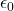is the permittivity of vacuum.

The value of this constant depends upon the medium that the charged objects are immersed in (most likely it is Air).In case of air, the value is approximately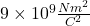And yes, this is a vector quantity (Force is always vector)

Direction: Force is repulsive between charges of same sign, while it is attractive between charges of opposite sign.

I have covered some examples in the below video

Electric field:

Electric field is defined as the force on a test charge (1C)

Due to a point charge q, Electric field E is defined as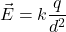Where d is the distance where electric field is to be measured.

Direction: For a positive charge, electric fields are always going out while for a negative charge, it is coming in. So, if we have a standalone positive charge, the electric fields will emerge out of it and terminate at infinity. While for a standalone negative charge, electric field lines would be coming from infinity and terminating on it.

I have covered some of its properties along with various other formulas of electric field due to line charge, circular wire, Ring etc. in this video

Stay tuned for further blog posts.

Struggling with one of your question? Post it down in the comment section.